• 交通指示灯模块描述由四种不同颜色的LED等组成。分别表示东部和西部,北部和南部方向。每10秒进行灯光切换，其中黄灯切换...交通指示灯电路板实物电路板截图: 所需器件组成: 交通指示灯电路板pcb 截图: BOM清单截图:
• 模拟十字路口的红绿灯以及数码管倒计时时间显示，51单片机代码附带proteus仿真电路原理图 直观实验现象
• ## 交通灯电路设计

千次阅读 2020-12-10 16:48:49
交通灯电路设计一、交通灯电路实现功能二、总体方案三、单元电路设计3.1状态转换电路3.2秒发生器3.3计时电路3.4数码管显示3.5复位四 、总体电路五、分析总结六、心得体会七、未来工作 一、交通灯电路实现功能 1.1...
交通灯电路设计一、交通灯电路实现功能二、总体方案三、单元电路设计3.1状态转换电路3.2秒发生器3.3计时电路3.4数码管显示3.5复位四	、总体电路图五、分析总结六、心得体会七、未来工作

一、交通灯电路实现功能
1.1主干道亮绿灯时，则支干道亮红灯，主干道通车，时间为 50 秒。
1.2转换时，绿灯先变为黄灯，5 秒后再变为红灯，同时另一方向道路的红灯转换为绿灯。
1.3主干道亮红灯时，则支干道亮绿灯，支干道通车，时间为 30 秒。
1.4用数码管指示绿灯和黄灯亮倒计时，其中绿灯在最后15秒才开始显示，以达到节能效果。
1.5黄灯闪烁，绿灯在最后5秒闪烁。
二、总体方案
首先进行逻辑抽象，用 G、Y、R 表示主干道的绿、黄、红灯，用 g、y、r 表示支干道的绿、黄、红灯，亮为‘‘1”。根据设计任务与要求，可知交通灯简单控制时序如图所示，图中系统运行共有四种状态：主干道绿灯亮(G=1)，50 秒后熄灭(G ↓)；主干道黄灯亮(Y=1)，5 秒后熄灭(Y↓)；支干道绿灯亮(g=1)，30 秒后熄灭(g↓)；支干道黄灯亮(y=1)，5 秒后熄灭(y↓)。之后又回到第一种状态：主干道绿灯亮……如此循环。图表 1.交通灯电压波形图图表 2.总体设计方案

可将系统分为三大功能模块：主电路、定时电路、控制电路部分。进一步细分，可将主电路分为主干道及支手道交通灯电路、译码显示电路；定时电路分为秒发生器、五十进制减法计数器、三十进制减法计数器、五进制加法计数器、计数器 CP 端控制电路；控制电路部分分为控制电路、复位开关。这样把总体方案划分为若干相对独立的单元。参考原理框图如图所示。
三、单元电路设计
3.1状态转换电路
在设计中要求实现四种状态的自动转换，首先把四种状态用数字表示出来，00-001010、01-100001、10-010001、11-001100。状态转换图和状态转化表如下：图表 3.状态转换图

表格 1.状态装换表

A
B
G
Y
R
g
y
r

0
0
0
0
0
1
0
1
0

1
0
1
1
0
0
0
0
1

2
1
0
0
1
0
0
0
1

3
1
1
0
0
1
1
0
0

本设计由两个JK触发器实现，由卡诺图化简，得到如下的公式，直接由与或非门连接即可$\left\{ \begin{array}{l} G=A'B\\ Y=AB'\\ R=A'B'+AB\\ g=AB\\ y=A'B'\\ r=A'B+AB'\\ \end{array} \right.$图表 4.状态转换模块电路

3.2秒发生器
秒发生器由555定时器连接成的多谐振电路组成，连接一定规格的电阻电容就可以产生方波脉冲。利用公式$T_1=R_3C_1\ln 2$
$T_2=R_4C_1\ln 2$得到 T=T1+T2=1s.图表 5.秒发生器模块电路图表 6.秒发生器波形图

3.3计时电路
计时电路由74LS190十进制加减计数器构成，分为5秒、30秒、50秒三组，且均为倒计时计数。如下图所示。图表 7.计时器模块电路

每一组的进位输出与JK触发器的输入时钟与或，从而达到将某一状态延时的作用。图表 8.计时器控制电路

3.4数码管显示
数码管显示模块由74LS47和共阳极数码管组成，共三组，分别是5秒黄灯倒计时、支路与主干路绿灯的15秒倒计时。数码管电路图如下：图表 9.数码显示电路

30秒、50秒绿灯倒计时的显示控制信号均是倒计时模块的输出信号经逻辑门电路形成的开关控制信号，SR锁存器将开关状态锁定输出给47芯片的灭灯输入端，具体电路如下：图表 10.数码管显示控制电路

3.5复位
复位电路分为上电复位和按键复位两部分。
上电复位由电容和电阻组成，当上电时，电容处于充电导通状态，所以输出高电平，通过反相器输出低电平，即复位信号。
按键复位由按压开关控制，按下开关，产生复位信号。图表 11.复位模块电路图

复位电路主要目的是将JK触发器的状态清零，减法计数器重新置位。
所以将置位信号分别输入到JK触发器的清零端与减法计数器的置位端即可。（其中减法计数器本身有一个置位信号且两个信号均是0有效，所以有与门）
四	、总体电路图图表 12.总体电路图

五、分析总结
竞争与冒险现象：
A、红绿灯显示时的竞争与冒险现象：红绿灯状态转换时会出现十分短暂的中间状态，经分析应为竞争与冒险的结果，其中产生竞争与冒险的原因是“反相器”的传输延迟。
B、数码显示时的竞争与冒险现象：如图，在数码显示信号“CB′A”到达之前，由于B′之前的“反相器”的传输延迟，波形图中的黄波迟于红波和绿波反相，出现短暂的“111”状态，经与门后的蓝波出现短暂的高电平。由于我们使用的时SR锁存器，在暂态后，显示状态经锁存，相当于显示信号提前两秒到达，最终出现的结果是数码管绿灯倒计时总是从“17秒”开始倒计时。我们的解决办法是在AC之前均添加两个“反相器”，经过调整之后，数码管倒计时从“15秒”开始。图表 13.因竞争与冒险产生的波形图表 14.消除竞争冒险后的波形

六、心得体会
从开始设计到全部完成历时两周，这中间出现了很多很多的问题，我们均一一解决，包括每个状态持续不同时间如何实现、绿灯倒计时和绿灯闪烁如何在15秒及5秒的时候才出现、555定时器仿真错误、电路中的竞争与冒险等等。收获一，电路不仅要正确无误，美观才更能体现设计的合理性，这样不仅能体现一个人的认真与耐心，更能为未来调试电路带来方便。收获二，电路出现问题后，可以使用示波器观察信号波形，找到问题后再分析出现的原因，寻找解决的办法，我们解决竞争与冒险的问题就是这样解决的。这样查错调试能力不是一时就能提高的，需要不断地尝试，动手多了才有经验。收获三，一个人的力量是有限的，团队合作才是王道，要懂得1+1>2的道理，团队之间相互配合，每个人发挥自己的优势能达到意想不到的效果。
七、未来工作
由于时间限制，我们的电路仍然存在一些不足，因此在未来我们需要做一定的改进，如
a．	红绿灯四个状态之间短暂的其他状态没有得到消除。因为使用的与非门过多，电路过于复杂，此处的竞争与冒险现象没有像数码显示信号处一样得到很好的解决。时间充裕的话可以耐心分析每一个信号暂态出现的原因，寻找更好的解决办法。
b．	由555定时器组成的秒发生器因为一些电阻电容参数等影响，电路仿真长时间后会出现仿真错误。因此研究一下555定时器组成的多谐振电路中电阻电容参数取值范围是有必要的。


展开全文经验分享
• 用altium10 制作，的 就是数字电路逻辑设计的 一些原理图
• 51单片机 交通灯 仿真与原理图 51单片机 交通灯 仿真与原理图51单片机 交通灯 仿真与原理图
• 交通灯的显示有很多方式，如十字路口、丁字路口等，而对于同一个路口又有很多不同的显示要求，比如十字路口，车辆如果只要东西和南北方向通行就很简单，而如果车子可以左右转弯的通行就比较复杂，本实验仅针对最简单...
• 主要是交通灯设计的总!但没有叙述，没有源程序，该有的都没有！
• 本系统采用MSC-51系列单片机ATSC51和可编程并行I/O接口芯片8255A为中心器件来设计交通灯控制器，实现了能根据实际车流量通过8051芯片的P1口设置红、绿灯燃亮时间的功能；红绿灯循环点亮，倒计时剩5秒时黄灯闪烁警示...
• 十字路口交通灯，附原理图和仿真，带程序。经验证，仿真真实有效。 附件部分截图如下:
• 这是一个简易的交通灯电路设计原理图，很简单就能看懂没希望大家有用的可以多下载看看，给点意见。
• 交通灯原理图，51单片机，带四组数码管，显示时间，每组两个
• 里面包好开题报告，任务书，制作详解，芯片资料，元件清单，论文，答辩问题，交通灯程序设计，原理图，PCB图，所需的任何软件都有。
• 交通灯控制器使用51单片机所实现的电路图，配合C语言代码实现控制，具体见我所上传的C语言代码
• 花了一个星期制作的交通灯仿真程序+原理图，通过数码管显示路口的时间，通过led显示红绿灯。@TOC ![#include<reg51.H>      // 对单片机的口进行了定义 #define uint unsigned int #...
花了一个星期制作的交通灯仿真程序+原理图，通过数码管显示路口的时间，通过led显示红绿灯。@[TOC]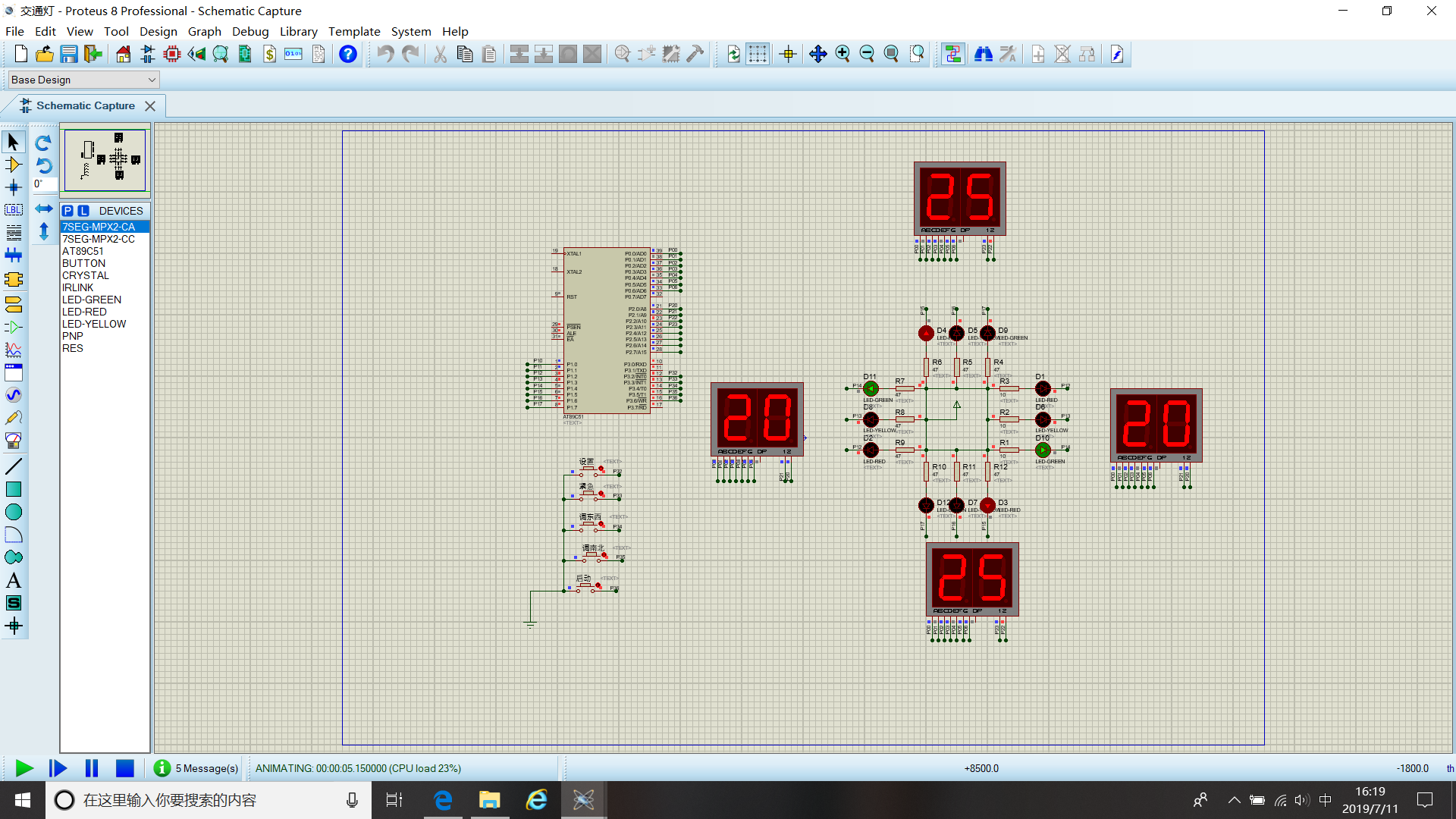#include<reg51.H>      // 对单片机的口进行了定义
#define uint unsigned int
#define uchar unsigned char
uchar code a={0x40,0x79,0x24,0x30,0x19,0x12,0x02,0x78,0x00,0x10};//段码组合,共阳极
uchar code b={0x01,0x02,0x04,0x08}; //位码组合
uchar code c={0xcc,0xd4,0x78,0xb8};/P1 绿红、黄红、红绿、红黄/
//12MHz
//*************************************************************************************
uchar NB=25,DX=30,NBG=25,DXG=15,H=5;    /数码管显示值设置/
uchar  i,k=0,cnt=0,j=0;
sbit K0=P3^4;      /支干道通行/
sbit K1=P3^5;      /主干道通行/
sbit K4=P3^6;      /返回/
sbit K3=P3^2;   /设置时间/
sbit K2=P3^3;   /紧急刹车/
void delay(uchar t);  /定义延时程序/
void key();    /定义键盘程序/
void display();   /定义显示程序/
void settime();  /定义时间设置显示程序/
//程序初始********************
void init(void)
{
TMOD=0x01;     /使用定时器0模式一/
TH0=0x3c;     /(65536-5000)/256/
TL0=0xb0;     /(65536-5000)%256/
IT0=1;      //开中断
ET0=1;
TR0=1;
EA=1;
EX0=1;
EX1=1;
P1=c[k];      //开启交通灯
}
//中断0处理程序**************
void int0(void) interrupt 0   // 通行时间设置
{
EA=0;
P1=0xd8;    /红灯全亮/
for(;?
{
settime();

if(K1==0)       /*P3^6=0设置主干道通行时间*/

{

delay(40);

if(K1==0)

{

while(!K1)

{settime();}

DXG++;    /*主干道通行时间加1*/

if(DXG==100)

DXG=0;

}

}

if(K0==0)        /*P3^5=0设置支干道通行时间*/

{

delay(40);

if(K0==0)

{

while(!K0)

{settime();}

NBG++;      /*支干道通行时间加1*/

if(NBG==100)

NBG=0;

}

}

if(K4==0)          /*P3^7=0返回*/

{

delay(40);

if(K4==0)

{

while(!K4)

{

}

k=0;P1=c[k];

NB=NBG,DX=NBG+H;

display();

EA=1;

break;

}

}

}

}
//***************中断1处理程序
void int1(void) interrupt 2      //紧急情况
{
    /*东西南北红灯亮*/

EA=0;
TR0=!TR0;           /停止计数/
for(;?           /主支干道显示全为0/
{
P1=0xd8,
P0=a;
P2=b;

delay(20);

P2=b;

delay(20);

P2=b;

delay(20);

P2=b;

delay(20);

if(K4==0)         /*返回*/

{
delay(20);
EA=1;
P1=c[k];       /返回紧急前状态开始计数/
TR0=!TR0;      /重启计数/
break;
}
}
}
void time1(void) interrupt 1      /交通灯控制程序/
{
TH0=0x3c;          /定时50ms/
TL0=0xb0;
cnt++;
if(cnt>=20)            /每2050ms=1s自减一*/
{
NB–;
DX–;
cnt=0;
if(NB0||DX0)
{
k++;
if(k>3)         /k取0,1,2,3/
k=0;
switch(k)
{
case 0:NB=NBG,DX=NBG+H;j=0;P1=c[k];break;   /*主干道通行显示绿红*/

case 1:NB=H;j=1;P1=c[k];break;        /*主干道黄灯闪烁*/

case 2:NB=DXG+H,DX=DXG;j=0;P1=c[k];break;   /*支干道通行显示红绿*/

case 3:DX=H;j=2;P1=c[k];break;        /*支干道黄灯闪烁*/

}
}
}
}
void delay(uchar t)    //延时程序延时0.1*nms
{
uchar i;
do
{
 for(i=0;i<20;i++)

;;;

} while(t–);
}
void settime()
{
P2=b,P0=a[(NBG+H)%10]; /*显示主干道通行时间  */

delay(20);

P2=b,P0=a[(NBG+H)/10];

delay(20);

P2=b,P0=a[(DXG+H)%10];  /*显示支干道通行时间*/

delay(20);

P2=b,P0=a[(DXG+H)/10];

delay(20);

}
void  key()    //键盘程序用KO K1模拟一道有车一道无车
{
 if(K1==0)        /*主干道有车支干道无车*/

{

delay(40);

if(K1==0)

{

while(!K1)

{

display();

}

k=0,P1=c[k];cnt=0;

NB=NBG, DX=NBG+H;

display();

}

}

if(K0==0)         /*支干道有车主干道无车*/

{

delay(40);

if(K0==0)

{

while(!K0)

{

display();

}

k=2,P1=c[k];cnt=0;

NB=DXG+H,DX=DXG;

display();

}

}
}
void display()       //显示程序
{
P2=b,P0=a[NB%10];      /由k的不同值进入确定显示数值/
delay(20);
P2=b,P0=a[NB/10];
delay(20);
P2=b,P0=a[DX%10];
delay(20);
P2=b,P0=a[DX/10];
delay(20);
}
void main(void)
{  //主程序
init();
for(;?
{
display();
key();
//黄灯闪烁程序
while(j==1)           /主干道黄灯闪烁/
   {

P1=0xdc;         /*给黄灯一高电平黄灯灭*/

for(i=83;i>0;i--){display();}      /*执行for循环延时*/

P1=c[k];         /*再给黄灯一低电平黄灯亮*/

for(i=83;i>0;i--){display();}

}
while(j==2)
     {              /*支干道黄灯闪烁*/

P1=0xf8;

for(i=83;i>0;i--){display();}

P1=c[k];

for(i=83;i>0;i--){display();}

}
}
}


展开全文• 　（4）了解交通灯控制电路工作原理。 　（5）熟悉交通灯控制电路的设计与制作。 2 设计要求 　（1）设计一个十字路口的交通灯控制电路，要求甲车道和乙车道两条交叉道路上的车辆交替运行，每次通行时间都设为25...
• 数电综合实验，采用基本芯片如138，192，153等
• 交通灯设计-控制电路图 数字电路课程设计所用 对弱电类学生有帮助
• 目录设计要求基于multisim对于交通灯的电路搭建1.1 交通灯电路的组成部分1.2 振荡脉冲模块1.3 计数模块及显示模块1.4 主控模块1.5 信号灯译码模块1.6 总电路及其仿真效果基于Quartus(verilog)对于交通灯的电路设计...
作者：王子齐
学校：电子科技大学
学号：2018151202014
设计目的：完成对于FGPA开发的基础练习，建立FPGA开发思想。
目录设计要求基于multisim对于交通灯的电路搭建1.1 交通灯电路的组成部分1.2 振荡脉冲模块1.3 计数模块及显示模块1.4 主控模块1.5 信号灯译码模块1.6 总电路及其仿真效果基于Quartus(verilog)对于交通灯的电路设计1.1 step by step1.1.1 首先给出状态转换表（此处状态编码为S1:000;S2:001:S3:010;S4:011;S5:100，即采用最简的状态编码形式）1.1.2	在1中已经得到了状态转换方程，下面给出状态输出表以便求出输出方程1.1.3 门级电路图。1.2 verilog 代码实现1.3 仿真效果总结
设计要求
设计并实现一个简单的十字路口交通灯控制电路。具体要求：以4个红色指示灯、4个绿色指示灯和4个黄色指示灯模拟路口东西南北4个方向的红绿黄交通灯。控制这些灯，使它们安下列规律亮灭。
1、东西方向绿灯亮，南北方向红灯亮。东西方向通车，时间30秒；
2、东西方向黄灯闪烁，南北方向红灯亮，时间2秒。
3、东西方向红灯亮，南北方向绿灯亮。南北方向通车，时间30秒；
4、东西方向红灯亮，南北方向黄灯闪烁，时间2秒。
5、返回1，继续运行。
要求在仿真软件中设计并运行，或设计实际电路调试运行，提交设计报告电子档，设计报告要求包括：设计方案、电路原理图、测试表格、分析结果总结，实现结果展示(要求用仿真结果截图或者实测照片截图展示实现效果)。
基于multisim对于交通灯的电路搭建
1.1 交通灯电路的组成部分
对于交通灯电路，其大致有以下几大模块：

模块
作用

振荡脉冲模块
产生信号源，使电路起振

计数及其显示模块
对于时间的一种显示，使得我们可以更好地了解交通灯的亮暗时间

主控模块
电路中最核心的部分，通过一定的数字逻辑关系对于最终的交通灯亮暗加以控制

信号灯译码模块
译码主控模块的控制信号，将其可视化

下面我们逐一进行讨论并给出相应multisim电路图
1.2 振荡脉冲模块
振荡脉冲模块事实上就是信号源产生模块，其主要功能就是为整个电路提高时钟脉冲，可以有多种方法去实现，直接使用时钟脉冲信号源或使用555定时器搭建多谐振荡器都可以实现相应目的，下面给出两种实现方法的电路图。
a)直接调用时钟脉冲（在multisim中搜索clock即可）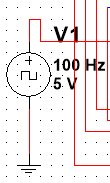b)使用555搭建的多谐振荡器（在multisim中搜索555即可）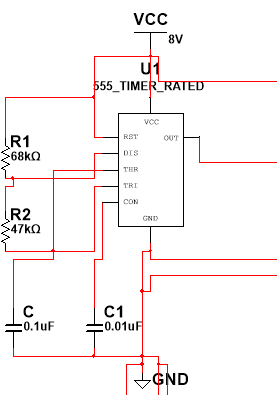如上图，此时的OUT等效于某个频率的时钟脉冲信号，通过调节R1,R2,C的值可以改变输出的频率，下面给出相应公式以便读者自行调节脉冲频率：
$t_{W1}=(R_{1}+R_{2})C$
$t_{W2}=R_{1}C$
$T=t_{W1}+t_{W2}$
1.3 计数模块及显示模块
计数电路是主控电路的输入源，通过计数电路的适当连接，可以得到我们希望的任意计数序列，而计数序列一方面可以当作时间在显示模块上得以可视化，另一方面又可以通过主控电路从而产生相应的控制信号。
对于显示电路，事实上，如果不考虑实际应用能力而只关心电路本身的性能，那么我们完全可以摒弃显示电路。但考虑在实际应用中，我们等待红绿灯时希望知道它的时间，所以在此加入显示电路。
译码电路采用计数器便可以实现，此处给出一种连接方法：
a) 基于74LS160构造的计数器（模64，因为一个交通灯周期为64个时间单位）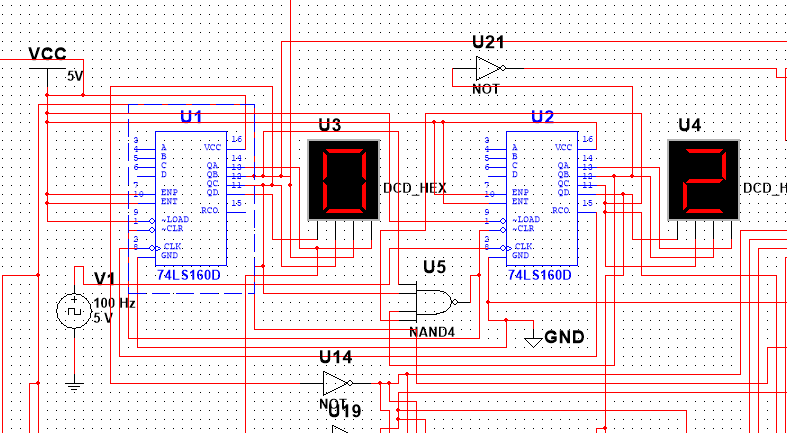级联方式非常简单，采用清零法级联即可。
1.4 主控模块
主控模块是对电路控制的核心力量，是由一些门电路组成的组合逻辑块。由于主控模块往往是电路设计中较为艰难的部分，所以下面给出详细的推导过程。我们令计数器U1的输出为 $Q_{A1}$ $Q_{B1}$ $Q_{C1}$ $Q_{D1}$ ,令计数器U2的输出为 $Q_{A2}$ $Q_{B2}$ $Q_{C2}$ $Q_{D2}$。令东西方向的黄灯，绿灯和红灯输出分别为$Y_{1}$  $G_{1}$  $R_{1}$，南北方向的黄灯，绿灯和红灯输出分别为$Y_{2}$  $G_{2}$  $R_{2}$。
计数器U1和U2级联形成模64计数器（0——63）。
根据上述设计要求，东西方向的黄灯只有在每次计数周期中低30—31个计数单位时亮起（前30S为东西方向绿灯亮起），故可列出如下真值表。(由于计数最大只计到63，故我们不关心U1的QD位）

输出项
$Q_{C1}$
$Q_{B1}$
$Q_{A1}$
$Q_{D2}$
$Q_{C2}$
$Q_{B2}$
$Q_{A2}$
$Y_{1}$
$G_{1}$
$R_{1}$
$Y_{2}$
$G_{2}$
$R_{2}$

0
0
0
0
0
0
0
0
0
1
0
0
0
1

1
0
0
0
0
0
0
1
0
1
0
0
0
1

2
0
0
0
0
0
1
0
0
1
0
0
0
1

3
0
0
0
0
0
1
1
0
1
0
0
0
1

4
0
0
0
0
1
0
0
0
1
0
0
0
1

5
0
0
0
0
1
0
1
0
1
0
0
0
1

6
0
0
0
0
1
1
0
0
1
0
0
0
1

7
0
0
0
0
1
1
1
0
1
0
0
0
1

8
0
0
0
1
0
0
0
0
1
0
0
0
1

9
0
0
0
1
0
0
1
0
1
0
0
0
1

10
0
0
1
0
0
0
0
0
1
0
0
0
1

11
0
0
1
0
0
0
1
0
1
0
0
0
1

12
0
0
1
0
0
1
0
0
1
0
0
0
1

13
0
0
1
0
0
1
1
0
1
0
0
0
1

14
0
0
0
1
1
0
0
0
1
0
0
0
1

15
0
0
1
0
1
0
1
0
1
0
0
0
1

16
0
0
1
0
1
1
0
0
1
0
0
0
1

17
0
0
1
0
1
1
1
0
1
0
0
0
1

18
0
0
1
1
0
0
0
0
1
0
0
0
1

19
0
0
1
1
0
0
1
0
1
0
0
0
1

20
0
1
0
0
0
0
0
0
1
0
0
0
1

21
0
1
0
0
0
0
1
0
1
0
0
0
1

22
0
1
0
0
0
1
0
0
1
0
0
0
1

23
0
1
0
0
0
1
1
0
1
0
0
0
1

24
0
1
0
0
1
0
0
0
1
0
0
0
1

25
0
1
0
0
1
0
1
0
1
0
0
0
1

26
0
1
0
0
1
1
0
0
1
0
0
0
1

27
0
1
0
0
1
1
1
0
1
0
0
0
1

28
0
1
0
1
0
0
0
0
1
0
0
0
1

29
0
1
0
1
0
0
1
0
1
0
0
0
1

30
0
1
1
0
0
0
0
1
0
0
0
0
0

31
0
1
1
0
0
0
1
1
0
0
0
0
1

32
0
1
1
0
0
1
0
0
0
1
0
1
0

33
0
1
1
0
0
1
1
0
0
1
0
1
0

34
0
1
1
0
1
0
0
0
0
1
0
1
0

35
0
1
1
0
1
0
1
0
0
1
0
1
0

36
0
1
1
0
1
1
0
0
0
1
0
1
0

37
0
1
1
0
1
1
1
0
0
1
0
1
0

38
0
1
1
1
0
0
0
0
0
1
0
1
0

39
0
1
1
1
0
0
1
0
0
1
0
1
0

40
1
0
0
0
0
0
0
0
0
1
0
1
0

41
1
0
0
0
0
0
1
0
0
1
0
1
0

42
1
0
0
0
0
1
0
0
0
1
0
1
0

43
1
0
0
0
0
1
1
0
0
1
0
1
0

44
1
0
0
0
1
0
0
0
0
1
0
1
0

45
1
0
0
0
1
0
1
0
0
1
0
1
0

46
1
0
0
0
1
1
0
0
0
1
0
1
0

47
1
0
0
0
1
1
1
0
0
1
0
1
0

48
1
0
0
1
0
0
0
0
0
1
0
1
0

49
1
0
0
1
0
0
1
0
0
1
0
1
0

50
1
0
1
0
0
0
0
0
0
1
0
1
0

51
1
0
1
0
0
0
1
0
0
1
0
1
0

52
1
0
1
0
0
1
0
0
0
1
0
1
0

53
1
0
1
0
0
1
1
0
0
1
0
1
0

54
1
0
1
0
1
0
0
0
0
1
0
1
0

55
1
0
1
0
1
0
1
0
0
1
0
1
0

56
1
0
1
0
1
1
0
0
0
1
0
1
0

57
1
0
1
0
1
1
1
0
0
1
0
1
0

58
1
0
1
1
0
0
0
0
0
1
0
1
0

59
1
0
1
1
0
0
1
0
0
1
0
1
0

60
1
1
0
0
0
0
0
0
0
1
0
1
0

61
1
1
0
0
0
0
1
0
0
1
0
1
0

62
1
1
0
0
0
1
0
0
0
1
1
0
0

63
1
1
0
0
0
1
1
0
0
1
1
0
0

根据上述真值表，可以采用卡诺图化简，下面给出详细推导过程。
(a)对于黄灯信号的卡诺图逻辑化简
对于东西方向的黄灯：
$Q_{C1} Q_{B1} Q{A1} = 000$ 时:$Y_{1}=0 , Y_{2}=0$
$Q_{C1} Q_{B1} Q{A1} = 001$ 时:$Y_{1}=0 , Y_{2}=0$
$Q_{C1} Q_{B1} Q{A1} = 010$ 时:$Y_{1}=0 , Y_{2}=0$
$Q_{C1} Q_{B1} Q{A1} = 011$ 时:$Y_{1}如下 , Y_{2}=0$

$Y_{1}/Q_{D2}$$Q_{C2}$
00
01
11
10

$Q_{B2}$ $Q_{A2}$
/
/
/
/

00
1
0
0
0

01
1
0
0
0

11
0
0
0
0

10
0
0
0
0

根据卡诺图化简可得到：
$Y_{1}=Q_{C1}^{'}Q_{B1}Q_{A1}Q_{D2}^ {'}Q_{B2}^{'}Q_{C2}^{'}$
同理，对于南北方向的黄灯：
$Q_{C1} Q_{B1} Q{A1} = 100$ 时:$Y_{1}=0 , Y_{2}=0$
$Q_{C1} Q_{B1} Q{A1} = 101$ 时:$Y_{1}=0 , Y_{2}=0$
$Q_{C1} Q_{B1} Q{A1} = 110$ 时:$Y_{1}=0 , Y_{2}如下：$

$Y_{1}/Q_{D2}$$Q_{C2}$
00
01
11
10

$Q_{B2}$ $Q_{A2}$
/
/
/
/

00
0
0
0
0

01
0
0
0
0

11
1
0
0
0

10
1
0
0
0

根据卡诺图化简可得到：
$Y_{2}=Q_{C1}Q_{B1}Q_{A1}^{'}Q_{D2}^ {'}Q_{B2}Q_{C2}^{'}$
(b)对于绿灯信号的逻辑推导
对于东西方向绿灯的处理：
$Q_{C1} Q_{B1} Q{A1} = 000$ 时:$G_{1}=1 , G_{2}=0$
$Q_{C1} Q_{B1} Q{A1} = 001$ 时:$G_{1}=1 , G_{2}=0$
$Q_{C1} Q_{B1} Q{A1} = 010$ 时:$G_{1}如下 , G_{2}=0$

$G_{1}/Q_{D2}$$Q_{C2}$
00
01
11
10

$Q_{B2}$ $Q_{A2}$
/
/
/
/

00
1
1
0
1

01
1
1
0
1

11
1
1
0
0

10
1
1
0
0

根据卡诺图化简可有：
$G_{1}=Q_{C1} ^ {'}Q_{B1} ^ {'}+Q_{C1} ^ {'}Q_{B1} +Q_{C1}^{'}Q_{B1}Q_{A1}^ {'}Q_{D2}Q_{B2} ^ {'}Q_{C2}^{'}$
对于南北方向绿灯的处理：
南北方向上的绿灯可以通过卡诺图化简，但更加便捷的方法是：当东西方向红灯亮且南北方向黄灯不亮时，则南北方向绿灯亮，基于此思想可以得到：
$G_{2}=（R_{1}+Y_{2}^{'})^{'}$
对于东西方向红灯的处理：
当南北方向绿灯或黄灯亮时，东西方向红灯亮，故有：
$R_{1}=G_{2}+Y_{2}$
对于南北方向红灯的处理：
当东西方向灯或黄灯亮时，南北方向红灯亮，故有：
$R_{1}=G_{2}+Y_{2}$
1.5 信号灯译码模块
当主控电路构建完成后，根据逻辑表达式连接到相应信号灯上即可。如下图：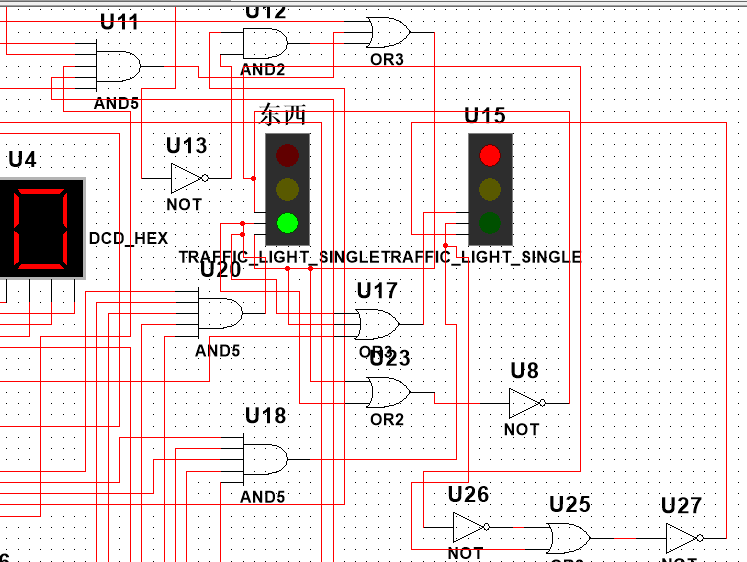1.6 总电路及其仿真效果
电路整体搭建效果如下图：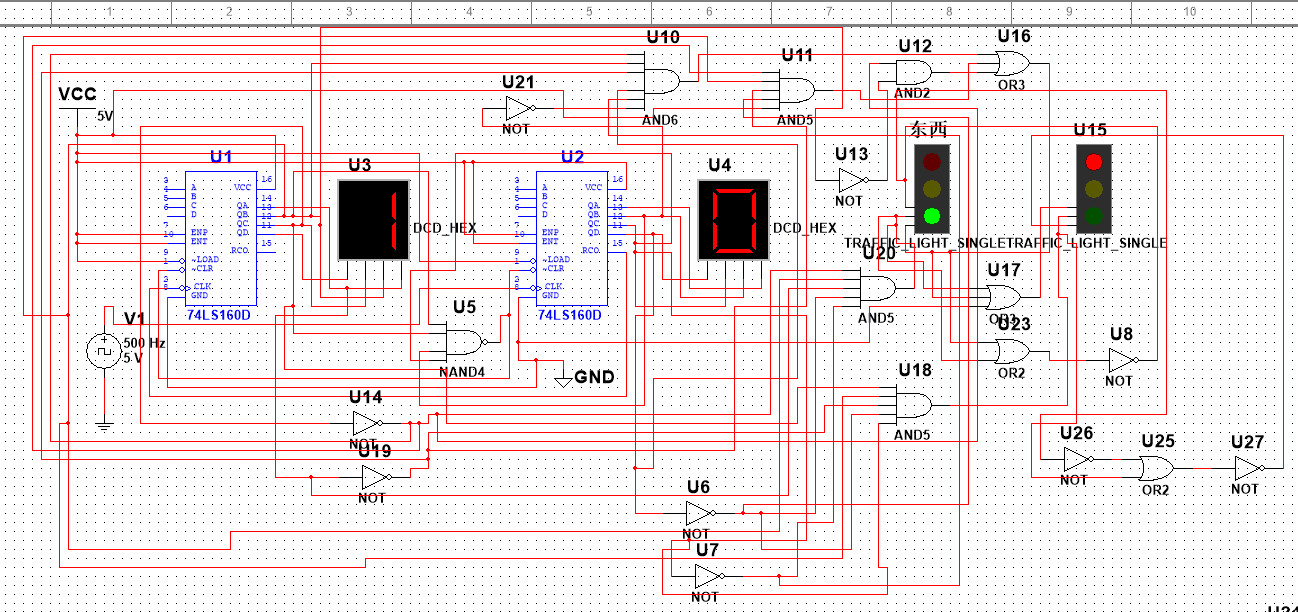相应仿真结果如下：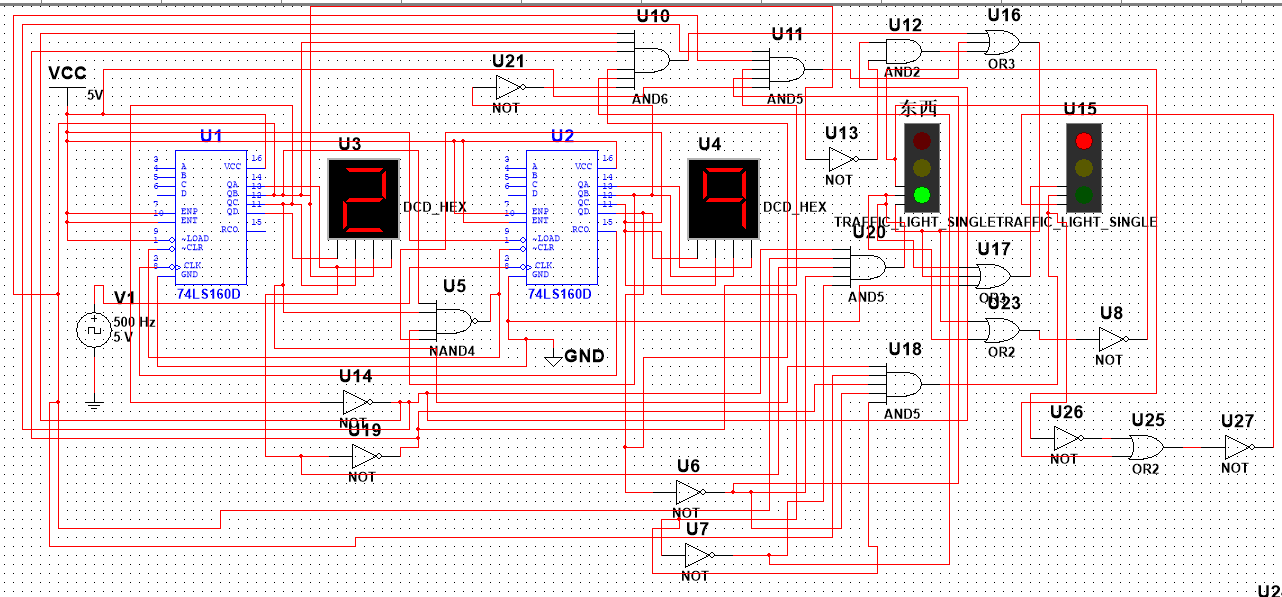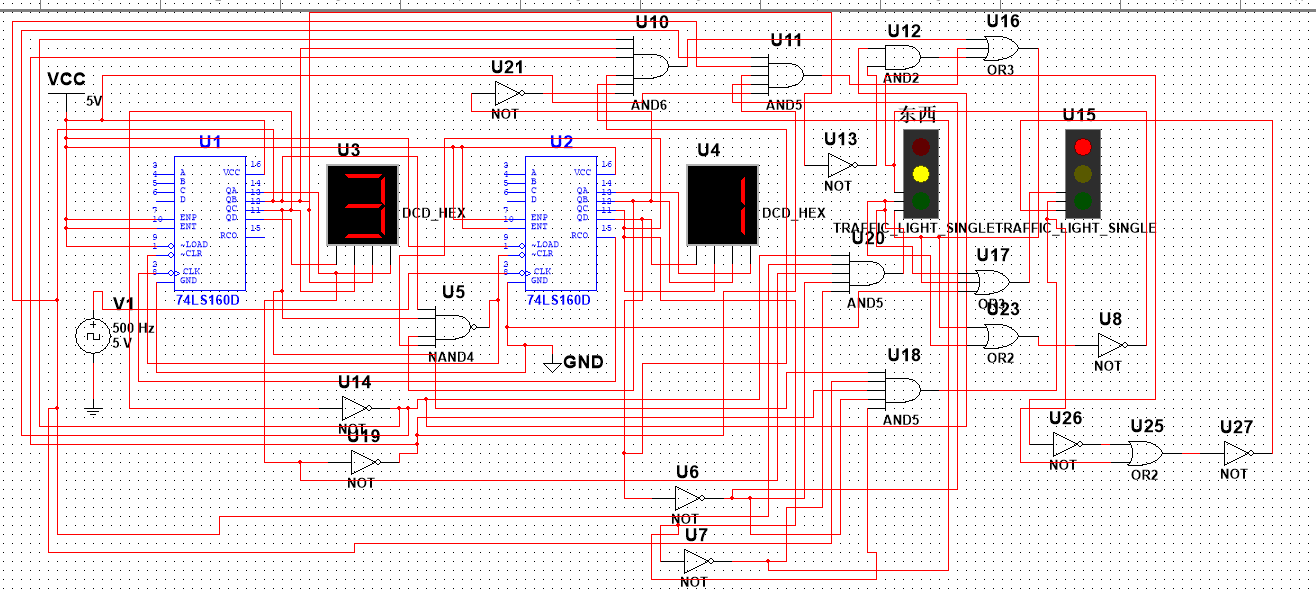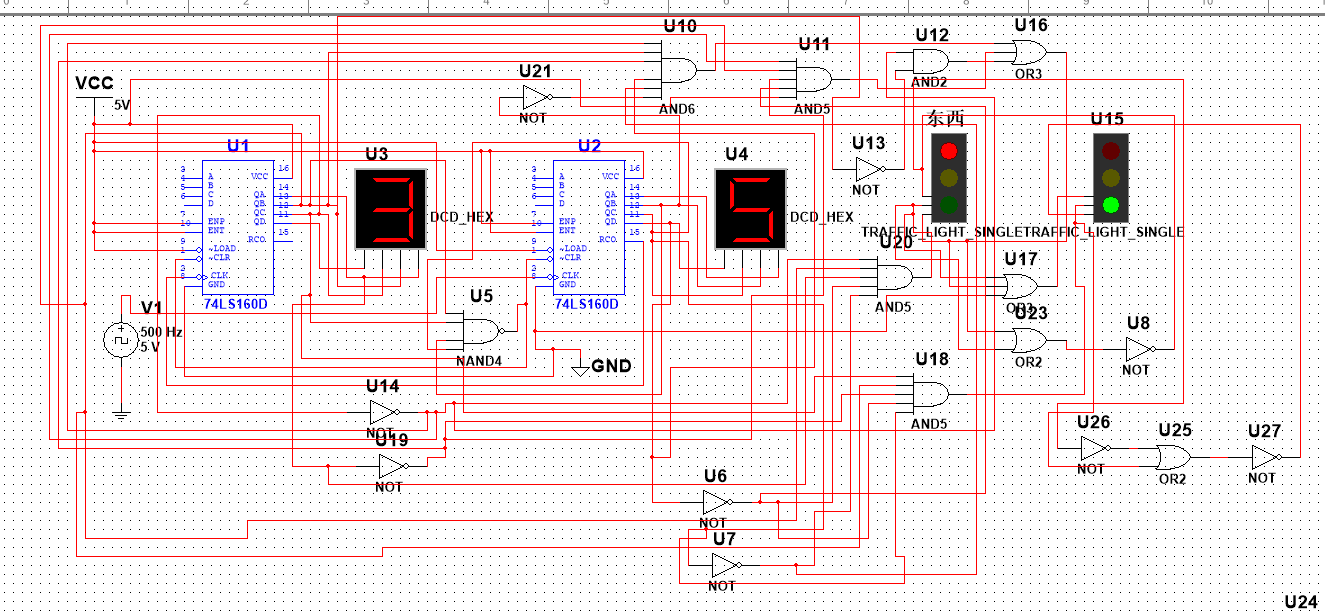基于Quartus(verilog)对于交通灯的电路设计
在介绍了multisim搭建电路实现交通灯的方法后，接下来介绍如何利用FPGA的思想去实现。
1.1 step by step
1.1.1 首先给出状态转换表（此处状态编码为S1:000;S2:001:S3:010;S4:011;S5:100，即采用最简的状态编码形式）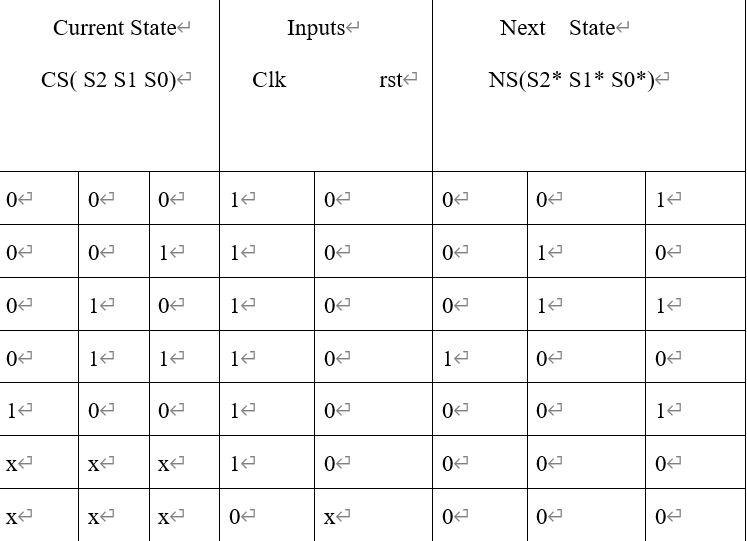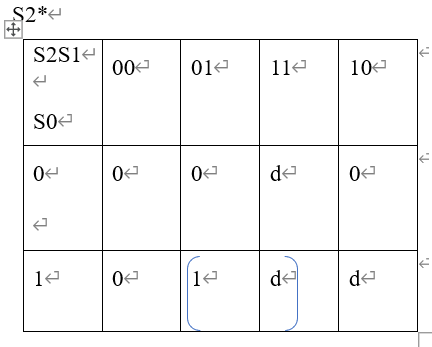（注：此处设计采用最小成本法）
根据卡诺图化简可得：S2*=S1S0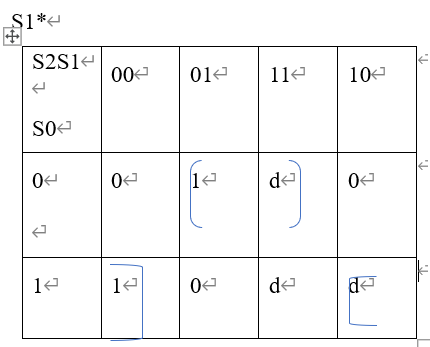（注：此处仍然采用最小成本法）
由卡诺图化简可得：S1*=S1S0’+S1’S0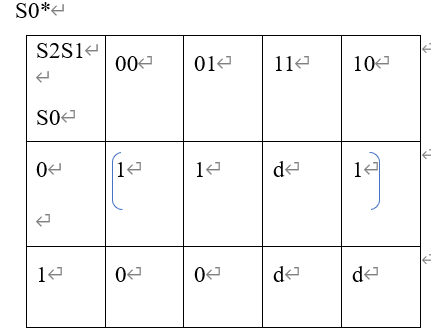(注：此处仍然采用最小成本法)
由卡诺图化简可得：S0*=S0’
1.1.2	在1中已经得到了状态转换方程，下面给出状态输出表以便求出输出方程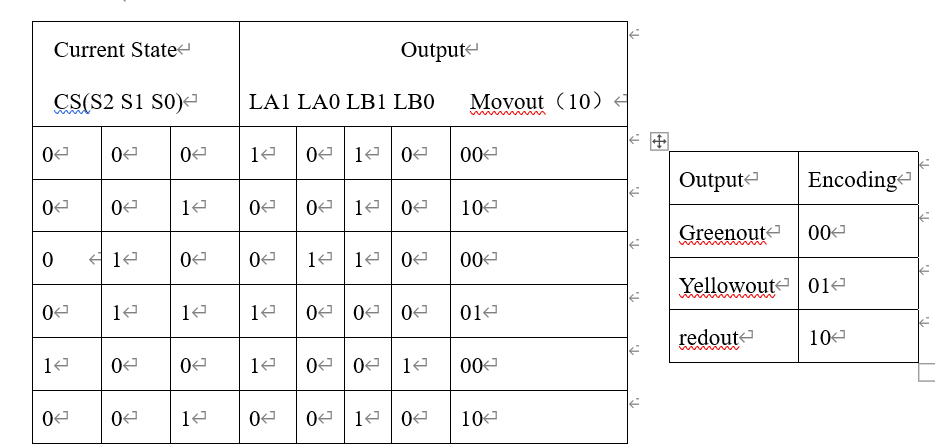(注：LA为东西方向的灯，LB为南北方向的灯)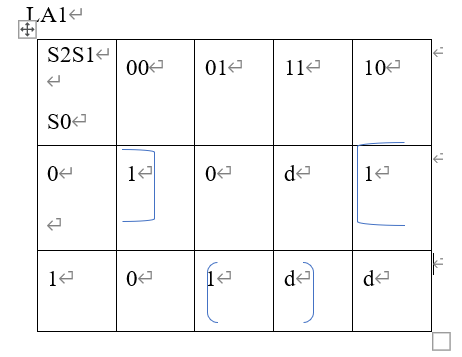（注：此处仍然采用最小成本法）  可有：LA1=S1’S0’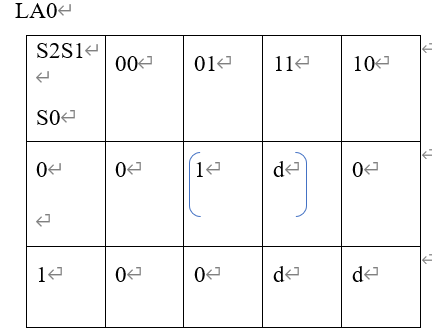（注：此处仍然采用最小成本法）
可有：LA0=S1S0’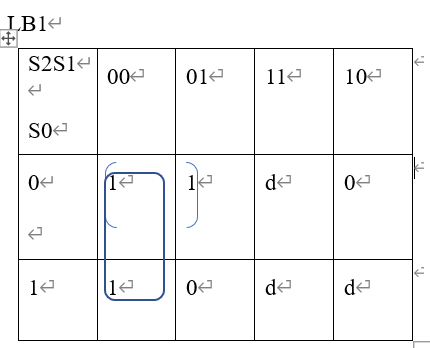（注：此处仍然采用最小成本法）
可有：LB1=S2’S0’+S2’S1’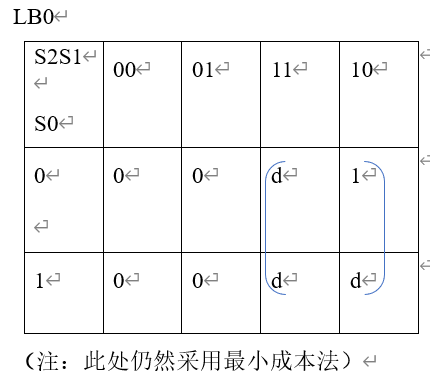（注：此处仍然采用最小成本法）
可有：LB0=S2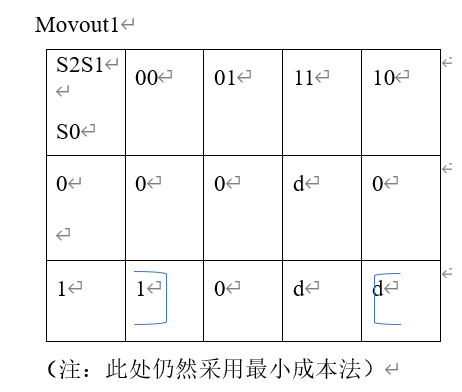（注：此处仍然采用最小成本法）
可有：Movout1=S1’S0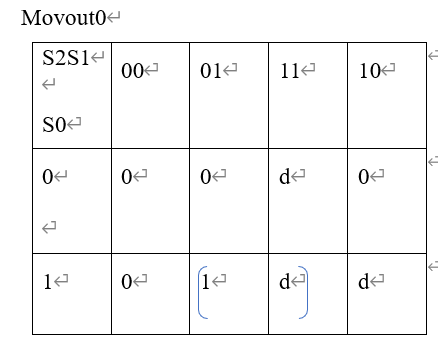（注：此处仍然采用最小成本法）
可有：Movout0=S1S0
1.1.3 门级电路图。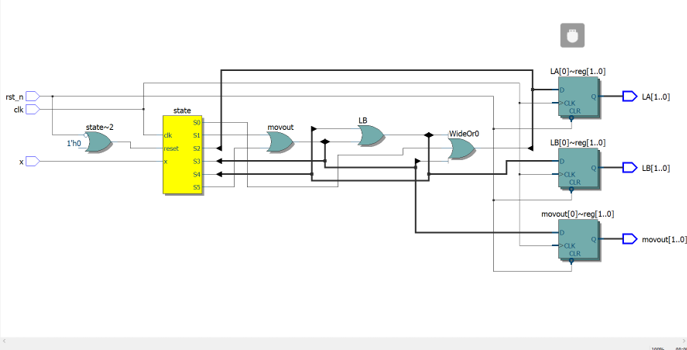1.2 verilog 代码实现
下面给出相应代码（verilog):
timescale 1 ps/ 1 ps       // 规定时间规模与时间精度
module trafficlight (        //建立模块
input clk,
input rst_n,            //端口说明
input x,
output  reg [1:0] LA,   // 指明LA为两位reg型输出
output  reg [1:0]LB,    // 指明LB为两位reg型输出
output  reg [1:0]movout  // 指明movout为两位reg型输出

);

reg [2 : 0] state;     //创立三位数组

parameter
S0=0,
S1=1,
S2=2,            //规定参数
S3=3,
S4=4,
S5=5;

always@(posedge clk or negedge rst_n)   //always块语句，括号中为敏感条件
Begin                         //begin-end语句
if(!rst_n)            //当rst_n为0时，执行下列语句
begin
LA=2'b00;         //   将2位二进制数00赋值给LA
LB=2'b00;
movout=2'b00;      //其余类似
state<=S0;        //进入S0状态
end
Else             //当rst_n为1时，执行下列语句
case(state)       //对于不同的状态有不同的情况
S0:             //若此时S0状态
begin
LA=2'b10;      // 将2位二进制数10赋值给LA
LB=2'b10;
movout=2'b00;
#3;    //延时3s
if(x==1)    //当x为1时
state<=S0;  //状态跳转到S0
Else     //当x为0时
state<=S1; //状态跳转到S1
end
S1:           //以下均类似
begin
LA=2'b00;
LB=2'b10;
movout=2'b10;
#30;
if(x==1)
state<=S1;
else
state<=S2;
end
S2:
begin
LA=2'b01;
LB=2'b10;
movout=2'b00;
#3;
if(x==1)
state<=S2;
else
state<=S3;
end
S3:
begin
LA=2'b10;
LB=2'b00;
movout=2'b01;
#30;
if(x==1)
state<=S3;
else
state<=S4;
end
S4:
begin
LA=2'b10;
LB=2'b01;
movout=2'b00;
#3;
if(x==1)
state<=S4;
else
state<=S5;
end
S5:
begin
LA=2'b00;
LB=2'b10;
movout=2'b10;
#30;
if(x==1)
state<=S5;
else
state<=S1;
end

default:      //出于严谨性的考虑，其余情况均执行下列操作
begin
LA=2'b00;
LB=2'b10;
movout=2'b10;
state<=S1;
end
endcase
end

endmodule


以及测试代码：
initial
begin
x=0;
clk=1;
rst_n=0;
#10 rst_n=1;
#200 \$stop;
end
always
begin
#1 clk=~clk;
end


1.3 仿真效果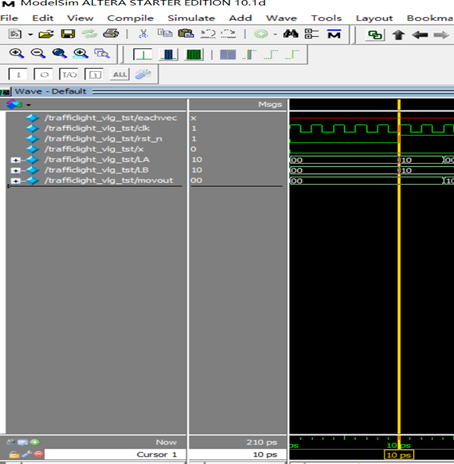在10个时间单位之后清零端被拉高系统开始有效运行，在起初三个时间单位内，可以看出LA是10，LB是10，movout是00，即初始状态为4个方向的红灯全亮，时间3个时间单位。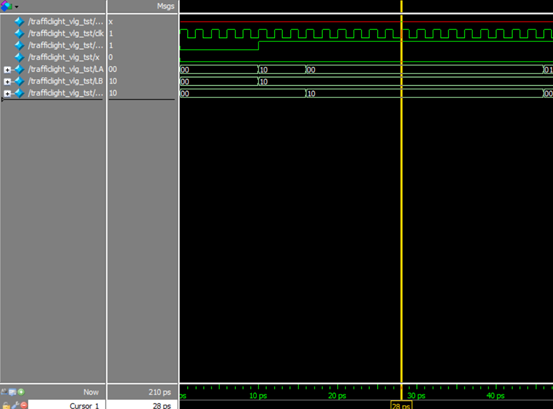在上述状态之后，进入下一状态，如上图，此时LA为00，LB为10，movout为10，即东、西方向绿灯亮，南、北方向红灯亮。东、西方向通车，持续30个时间单位，符合设计要求。
其余分析类似。
总结
在进行电路设计时，应该有化繁为简的思想。对于复杂电路，我们可以将其分解为相应功能模块，逐一实现，最终级联即可。
未经允许请勿转载
欢迎交流


展开全文fpga
• 2．掌握交通灯的亮灭规律和控制器的工作原理。 3．进一步熟悉 VHDL 语言编程，了解实际设计中的优化方案。 二、实验内容 1．实验前完成交通灯控制电路程序的编写。内容是设计一个简单的交通灯控制器，交通灯显示用...
交通灯控制电路设计
一、实验目的
1．掌握一般状态机的设计与应用。
2．掌握交通灯的亮灭规律和控制器的工作原理。
3．进一步熟悉
VHDL 语言编程，了解实际设计中的优化方案。
二、实验内容
1．实验前完成交通灯控制电路程序的编写。内容是设计一个简单的交通灯控制器，交通灯显示用实验箱的交通灯模块来显示。系统时钟选择时钟模块的 1Hz时钟，黄灯闪烁时钟要求为1Hz，红灯15s，黄灯 5s，绿灯15s。系统中用 CPU板上的复位按键进行复位。
2．本实验需要用到实验箱上交通灯模块中的发光二极管，即红、黄、绿各三个。其交通灯的亮灭规律为：初始态是两个路口的红灯全亮，之后东西路口的绿灯亮，南北路口的红灯亮，东西方向通车，延时一段时间后，东西路口绿灯灭，黄灯开始闪烁。闪烁若干次后，东西路口红灯亮，而同时南北路口的绿灯亮，南北方向开始通车，延时一段时间后，南北路口的绿灯灭，黄灯开始闪烁。闪烁若干次后，再切换到东西路口方向，重复上述过程。
3．完成VHDL程序的编译并仿真。仿真无误后，对交通灯模块的 LED对应的FPGA 引脚进行管脚绑定，然后再重新编译一次。用下载电缆通过 JTAG接口将对应的sof 文件下载到FPGA中。
4．功能选择位 M[3…0]状态均为0111，则16位数据线和交通灯控制电路相连接。CLK_1，即对应 IO3（用导线连接
实验代码


LIBRARY IEEE;

USE IEEE.STD_LOGIC_1164.ALL;

USE IEEE.STD_LOGIC_UNSIGNED.ALL;

ENTITY traffic_light is

GENERIC( green1:integer:=15;--定义东西绿灯亮15S

yellow1 :integer:=5 ;--定义东西黄灯亮5S

green2:integer:=15;--定义南北绿灯亮15S

yellow2 :integer:=5);--定义南北黄灯亮5S

PORT(
CLK,RST: IN STD_LOGIC;

w_r,w_y,w_g,e_r,e_y,e_g,n_r,n_y,n_g,s_r,s_y,s_g: out STD_LOGIC

);

End traffic_light;

ARCHITECTURE rtl of traffic_light is

Type states is (st0,st1,st2,st3,st4);--定义控制器各种状态

Signal state:states:=st4; --初始化状态

Signal cnt:integer range 0 to 15;--定义计数器

Signal cnt_enb:std_logic:='0'; ---初始化计数器使能信号

begin

AA:Process(clk,rst)

Begin

if (rst='1') then state<=st4;
cnt<=1;

Elsif (clk'event and clk='1') then

if (cnt_enb='1') then
cnt<=cnt+1;--计数器计数

else cnt<=1;  end if;

Case state is

when st4=>state<=st0;

when st0=>if (cnt=green1 ) then
state<=st1; --东西绿灯时间到

Else state<=st0; End
if;

when st1=>if (cnt=yellow1 ) then
state<=st2; --东西黄灯时间到

Else state<=st1; End
if;

when st2=>if (cnt=green2 ) then
state<=st3; --南北绿灯时间到

Else state<=st2; End
if;

when st3=>if (cnt=yellow2 ) then
state<=st0; --南北黄灯时间到

Else state<=st3; End
if;

End case;

End if;

End
process AA;

BB:Process(state)

Begin

Case
state is

when
st0=> w_r<='0'; w_y<='0';
w_g<='1';e_r<='0';e_y<='0';e_g<='1';--东西绿灯亮

s_r<='1'; s_y<='0';
s_g<='0';n_r<='1';n_y<='0';n_g<='0';Cnt_enb<='1';--南北红灯亮

if (cnt=green1) then cnt_enb<='0'; end
if;

when
st1=> w_r<='0'; w_y<='1';
w_g<='0';e_r<='0';e_y<='1';e_g<='0'; --东西黄灯亮

s_r<='1'; s_y<='0';
s_g<='0';n_r<='1';n_y<='0';n_g<='0';Cnt_enb<='1'; --南北红灯亮

if (cnt=yellow1) then cnt_enb<='0'; end
if;

when
st2=> w_r<='1'; w_y<='0'; w_g<='0';e_r<='1';e_y<='0';e_g<='0';
--东西红灯亮

s_r<='0'; s_y<='0';
s_g<='1';n_r<='0';n_y<='0';n_g<='1';Cnt_enb<='1';--南北绿灯亮

if (cnt=green2) then cnt_enb<='0'; end
if;

when
st3=> w_r<='1'; w_y<='0';
w_g<='0';e_r<='1';e_y<='0';e_g<='0';--东西红灯亮

s_r<='0'; s_y<='1';
s_g<='0';n_r<='0';n_y<='1';n_g<='0';Cnt_enb<='1';--南北黄灯亮

if (cnt=yellow2) then cnt_enb<='0'; end
if;

when st4=> w_r<='1'; w_y<='0';
w_g<='0';e_r<='1';e_y<='0';e_g<='0'; --东西红灯亮

s_r<='1'; s_y<='0';
s_g<='0';n_r<='1';n_y<='0';n_g<='0';Cnt_enb<='1';--南北红灯亮

if(rst='1')then cnt_enb<='0';end if;

End case;

End
process BB;

End rtl;




展开全文• 设计一个交通灯控制器，掌握倒计时计数器、译码器等的工作原理和设计方法。 要 求： 　1．设计一个十字路口的的交通灯系统，要求甲车道和乙车道两条交叉道路口的车辆交替运行，每次通过时间都设为25秒。 2．要求...
• 基于单片机80C51 C语言制作LED模拟交通灯，包含Proteus仿真原理图及Keil C51源码。 资源来源网络，仅供学习参考，请勿用于商业用途。
• 1、设计一个十字路口的交通灯控制电路，要求南北方向（主干道）车道和东西方向（支 干道）车道两条交叉道路上的车辆交替运行，主支干道每次通行时间都设为25秒、。 2、在绿灯转为红灯时，要求黄灯先亮5秒钟，才能...
• 交通灯控制电路，源程序，at89s52单片机，原理图，code，
• 这是一次的课程设计,实物完成正常,效果一样.有什么不懂的,可以留言,讨论.
• 十字路口交通灯控制设计(附电路图及源代码)《计算机控制技术》课程设计报告课题名称 交通灯控制设计专 业班 级学生姓名学 号指导教师201*年 6 月 8日目录TOC \o "1-1" \h \u HYPERLINK \l _Toc16316 一、总体方案...
• 该设计与单片机专业知识结合紧密，结合实际情况阐述了交通灯控制系统的工作原理，给出了一种简单低成本城市交通灯控制系统的硬件及软件设计方案、各个路口交通灯的状态循环显示，并对程序流程进行详细讲解分析。...
•  摘要：分析了现代城市交通控制与管理问题的现状，结合城乡交通的实际情况阐述了交通灯控制系统的工作原理，给出了一种简单实用的城市交通灯控制系统的硬件电路设计方案。  关键词：交通控制 交通灯 时间发生......